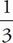# SAT Math Multiple Choice Question 19: Answer and Explanation

### Test Information

Question: 19

5. How many solutions exist to the equation |x| = |2x - 1| ?

• A. 0
• B. 1
• C. 2
• D. 3

C If |x| = |2x - 1|, either x = 2x - 1 or -x = 2x - 1. The solutions to these equations are 1 and, respectively. However, the only thing you need to recognize is that the equation has two different solutions to establish that the answer is (C).